Unit Weight Determination - Water Displacement Method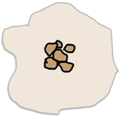Water displacement method is another method to determine the unit weight of soil sample brought from the field. This method is suitable only for the cohesive soils because we need a soil sample in the form of a lump.

Cohesive soils are those whose particles adhere to each other.From the field we take out a small lump of soil from the ground and trim it down to have some regular shape. This trimmed sample is weighed and weight noted down as W1.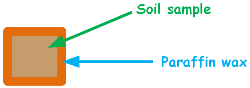Now we will determine the volume of this sample by dipping it into the water. But soil will disintegrate if it comes in contact with water. So to protect the disintegration, sample is coated with the thin layer of paraffin wax. It has been shown thicker here for better visibility.

This coated sample is weighed again and weight is noted as W2.

We have weight of sample W1.

Weight of sample and wax W2.

Hence weight of wax Wwax would be W2 minus W1.

Here volume of wax can be determined by unit weight formula as this.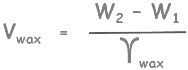This is the volume of wax used to cover the soil sample.Next we take a container with overflow management attached to it. Overflow management means if we keep on filling this container with some liquid even after reaching its full capacity, the extra liquid should not just spill over around but with a proper management it should get collected.

Now fill this container up to the brim with water. Gradually lower the coated sample into it. Because of it water level will rise and overflow water will be collected into the measuring jar.

Lets say measured volume is Vw. This is the volume of water displaced by coated soil sample.

Now as we know volume of displaced liquid is equal to the volume of object which displaced it hence volume of collected water is equal to the volume of coated sample. now this displaced liquid volume is volume of soil sample plus volume of wax.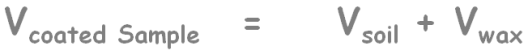We have already calculated volume of wax as this.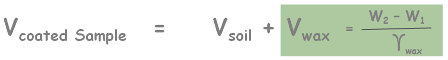Which implies that volume of sample is this.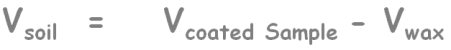Now by simply applying unit weight formula, we can calculate unit weight of soil sample which will be equal to weight of soil sample only divided by its volume.Now if we take a small sample from this lump of soil and determine the water content of the soil using any of the water content determination methods, then we can also calculate dry unit weight of this soil using this formula.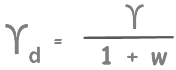Here gamma is unit weight of soil that we determined in this test and w is water content.

Tags : unit weight, soil test

Published on :2019-09-06